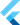# Getters and setters

on Saturday, 18th of July, 2020

Getters and setters are special methods that provide explicit read and write access to an object's properties.

### Getters

Getters are often useful to set computed properties. In other words, instance variables that rely on other, non-constant variables.

``````class Rectangle {
final int width, height;

Rectangle(this.width, this.height);

// This computed property is treated like a function
// that returns a value.
int get area => width * height;
}``````

### Setters

Setters are similar to getters in that they're syntactic-sugar for methods. But, setters don't provide read-access to a variable. They're used to set the value of a variable and nothing else.

These are often useful when you want to execute some code in response to a variable being assigned a value, or when a properties value needs to be computed when set.

``````class Rectangle {
final int width, height;

Rectangle(this.width, this.height);

int get area => width * height;

// Use a private variable to
// expose the value with a getter;
Point _center;
Point get center => _center;
set center(Point origin) {
_center = Point(
origin.x + width / 2,
origin.y + height / 2,
);
}
}

void main() {
var rectangle = Rectangle(12,6);
print(rectangle.area);
// The setter will calculate the center based on what we tell it is the
// _origin_ (top left corner) of the rectangle on a plot.
// in this case, we're setting the origin at (4,4).
rectangle.center = Point(4,4);
print(rectangle.cernter);
}``````